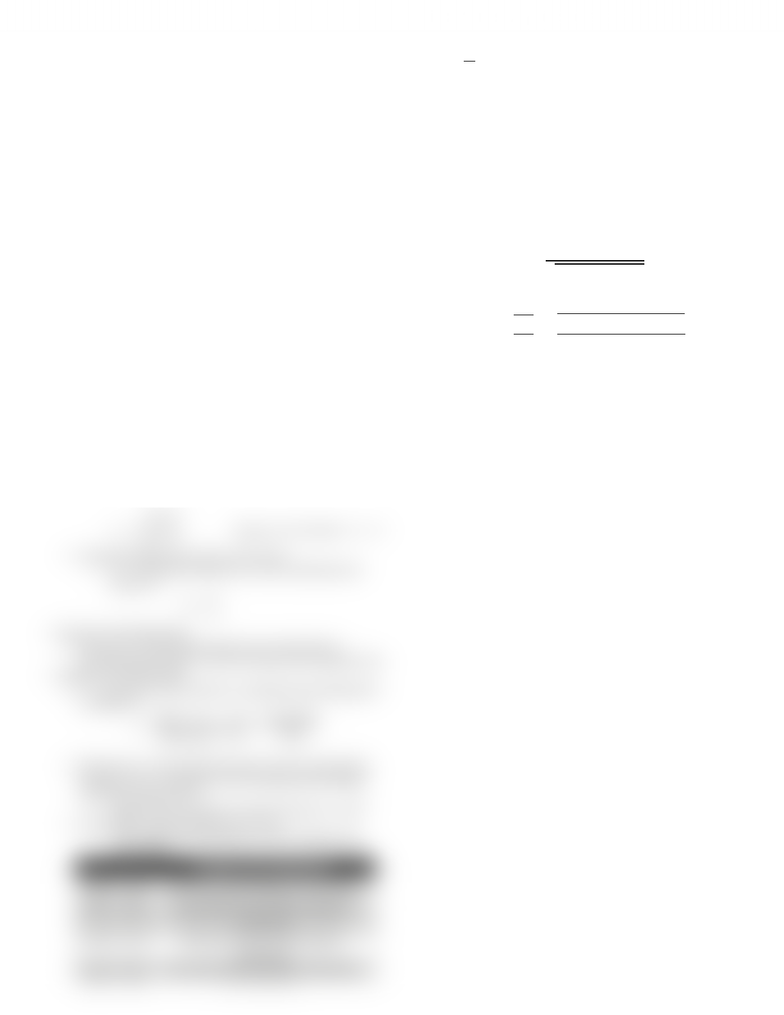Class Notes (1,000,000)
CA (610,000)
UTSG (50,000)
SOC (3,000)
Lecture

SOC202H1 Lecture Notes - Linear Regression, Null Hypothesis, Statistic

Department
Sociology
Course Code
SOC202H1
Professor
Scott Schieman

This preview shows half of the first page. to view the full 1 pages of the document.CH15 BIVARIATE CORRELATIONSHIP AND REGRESSION: HYPOTHESIS TESTING
Hypothesis Test
o First compute
and
o Then compute SCP and TSS
o Find Pearson’s r correlation coefficient, regression coefficient b
o Then use
and
and b to compute a
o Then specify regression line equation, plot it w/ lowest & highest X
values on the scatterplot
6 steps of statistical inference and 4 aspects of a relationship
o Requirements:
There is one representative sample from a single population
There are two interval/ratio variables
There are no restrictions on sample size, but generally, the larger
the n the better
Scatterplot of coordinates of the two variables fits a linear
pattern
o Existence of a Relationship
Does a linear relationship between X and Y truly exist in the
population or is the linear pattern in this sample the result of
sampling error?
ρ (rho) corresponding parameter of Pearson’s r statistic
measures the tightness of fit of coordinates around the
regression line for the population
if there’s no relationship in the population then ρ = 0
Pearson’s r will = 0 give or take sampling error
The effect of the hypothesis test is the difference between
observed sample statistic and the expected parameter when the
null hypothesis is true, hence:

Where: and
testing the significance of Pearson’s r bivariate correlation
coefficient: t-Test
Pearson’s r will center on zero as an approximately normal
t-distribution

Calculating Standard Error from a rho of zero
= estimated standard error of the t-distribution for
Pearson’s r
o Direction of the Relationship
Direction of a relationships between two interval/ratio is
ascertained by the sign of r and b, the slope of the regression line
o Strength of the Relationship
= proportion of the variation in Y explained by knowing that it
is related to X




When there’s a strong relationship btwn the two interval/ratio
variables, the X Y coordinates on the scatterplot will fit tightly
around the regression line
Tighter the fit, the larger the value of Pearson’s r and r2
For strength of relationship focus on r2 not r
r encourages an overestimation of the strength of the
relationships
r
r2
Strength of the Relationship
±1.00
1.00
Perfect positive/negative relationship
±0.90
0.81
Very strong positive/negative relationship
±0.70
0.49
Moderately strong positive/negative
relationship
±0.40
0.16
Moderately weak positive/negative
relationship
±0.10
0.01
Very weak positive/negative relationship
±0.00
0.00
No relationship
o t-statistic of the sample?

Larger slope and smaller standard error yield stronger evidence
against the null hypothesis
Standard Error of the slope ()
Estimates the degree of sample-to-sample variation if
regression slopes were calculated from many random
samples of size n
A small standard error implies a higher likelihood that
most of the sample slopes would be near the true
population slope
Larger standard error implies that the regression
coefficient estimate may not accurately reflect the true
relationship between X and Y in the population

Standard deviation of the residual ()



Careful Interpretations of Correlation and Regression Statistics
o Correlation apply to a population not an individual
o Careful interpretation of the slope, b
o Distinguishing statistical significance from practical significance
Tabular Presentation: Correlation tables